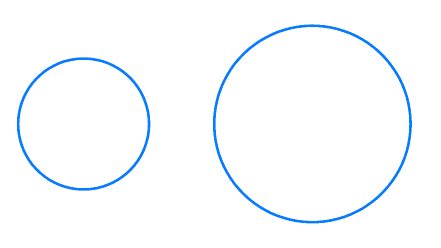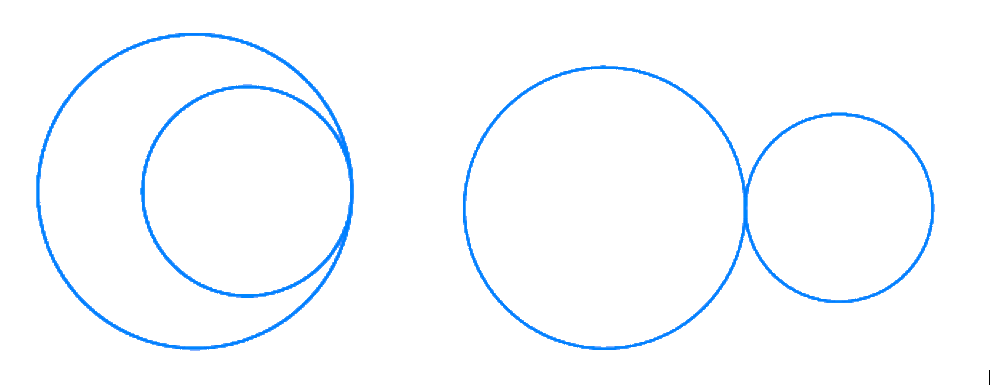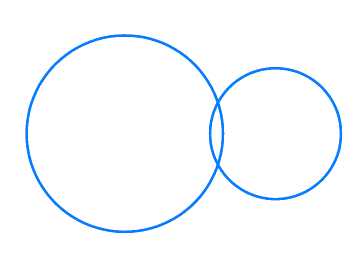# Ex.10.3 Q1 Circles Solution - NCERT Maths Class 9

Go back to  'Ex.10.3'

## Question

Draw different pairs of circles. How many points does each pair have in common? What is the maximum number of common points?

Video Solution
Circles
Ex 10.3 | Question 1

## Text Solution

What is known?

Pair of circles.

What is unknown?

No. of points does each pair have in common and what can be maximum common points

Reasoning:

We can draw no. of possible pairs and can observe common points in each case

Steps:

In this there are no common points.In this there is only one common point.In this there are two common points.In a situation where two congruent circles are superimposed on each other, it can be understood to as if we are drawing the same circle two times.

It is therefore concluded, there can be a maximum of two points for different pairs of circles.

Video Solution
Circles
Ex 10.3 | Question 1

Learn from the best math teachers and top your exams

• Live one on one classroom and doubt clearing
• Practice worksheets in and after class for conceptual clarity
• Personalized curriculum to keep up with school